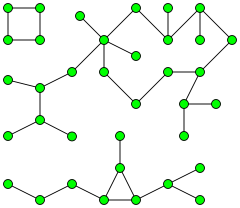# Coding Puzzle in F#: Find the Number of Islands

Here’s a programming puzzle. Given 2D matrix of 0’s and 1’s, find the number of islands. A group of connected 1’s forms an island. For example, the below matrix contains 5 islands

``````Input : mat = {{1, 1, 0, 0, 0},
{0, 1, 0, 0, 1},
{1, 0, 0, 1, 1},
{0, 0, 0, 0, 0},
{1, 0, 1, 0, 1}}
Output : 5
``````

A typical solution to this problem will be implemented in C++, Java or C# and will involve a loop to iterate through the matrix, and another loop or recursion to traverse islands. The traversal progress will be tracked in an auxiliary mutable array, denoting the visited nodes. An example of such solution (and the definition of the problem above) can be found here.

I want to give an example of solution done in F#, with generic immutable data structures and pure functions.

## Graph Traversal

First of all, this puzzle is a variation of the standard problem: Counting number of connected components in a graph.I will start my implementation with a graph traversal implementation, and then we will apply it to the 2D matrix at hand.

The graph is defined by the following type:

``````type Graph<'a> = {
Nodes: seq<'a>
Neighbours: 'a -> seq<'a>
}
``````

It is a record type with two fields: a sequence of all nodes, and a function to get neighbour nodes for a given node. The type of the node is generic: I’ll use numbers for our example, but `Graph` type doesn’t care much.

The traversal plan is the following:

1. Go through the sequence of graph nodes.

2. Keep two accumulator data structures: the list of disjoint sub-graphs (sets of nodes connected to each other) and the set of visited nodes. Both are empty at the beginning.

3. If the current node is not in the visited set, recursively traverse all neighbours to find the current connected component.

4. The connected component traversal is the Depth-First Search, each node is added to both current set and total visited set.

Let’s start the implementation from inside out. The following recursive function adds a node to the accumulated sets and calls itself for non-visited neighbours:

``````let rec visitNode accumulator visited node =
let newAccumulator = Set.add node accumulator
let newVisited = Set.add node visited

graph.Neighbours node
|> Seq.filter (fun n -> Set.contains n newVisited |> not)
|> Seq.fold (fun (acc, vis) n -> visitNode acc vis n) (newAccumulator, newVisited)
``````

The type of this function is `Set<'a> -> Set<'a> -> 'a -> Set<'a> * Set<'a>`.

Step 3 is implemented with `visitComponent` function:

``````let visitComponent (sets, visited) node =
if Set.contains node visited
then sets, visited
else
let newIsland, newVisited = visitNode Set.empty visited node
newIsland :: sets, newVisited
``````

Now, the graph traversal is just a `fold` of graph nodes with `visitComponent` function.

``````module Graph =
let findConnectedComponents graph =
graph.Nodes
|> Seq.fold visitComponent ([], Set.empty)
|> fst
``````

This is the only public function of our graph API, available for the client applications. The `visitNode` and `visitComponent` are defined as local functions underneath (and they close over the graph value).

## 2D Matrix

Now, let’s forget about the graphs for a second and model the 2D matrix of integers. The type definition is simple, it’s just an alias for the array:

``````type Matrix2D = int[,]
``````

Now, we need to be able to traverse the matrix, i.e. iterate through all elements and find the neighbours of each element.

The implementation below is mostly busy validating the boundaries of the array. The neighbours of a cell are up to 8 cells around it, diagonal elements included.

``````module Matrix2D =
let allCells (mx: Matrix2D) = seq {
for x in [0 .. Array2D.length1 mx - 1] do
for y in [0 .. Array2D.length2 mx - 1] -> x, y
}

let neighbours (mx: Matrix2D) (x,y) =
Seq.crossproduct [x-1 .. x+1] [y-1 .. y+1]
|> Seq.filter (fun (i, j) -> i >= 0 && j >= 0
&& i < Array2D.length1 mx
&& j < Array2D.length2 mx)
|> Seq.filter (fun (i, j) -> i <> x || j <> y)
``````

## Putting It All Together

Now we are all set to solve the puzzle. Here is our input array:

``````let mat = array2D
[| [|1; 1; 0; 0; 0|];
[|0; 1; 0; 0; 1|];
[|1; 0; 0; 1; 1|];
[|0; 0; 0; 0; 0|];
[|1; 0; 1; 0; 1|]
|]
``````

We need a function to define if a given cell is a piece of an island:

``````let isNode (x, y) = mat.[x, y] = 1
``````

And here is the essence of the solution - our graph definition. Both `Nodes` and `Neightbours` are matrix cells filtered to contain 1’s.

``````let graph = {
Nodes = Matrix2D.allCells mat |> Seq.filter isNode
Neighbours = Matrix2D.neighbours mat >> Seq.filter isNode
}
``````

The result is calculated with one-liner:

``````graph |> Graph.findConnectedComponents |> List.length
``````

## Conclusion

The implementation above represents my attempt to solve in a functional way the puzzle which is normally solved in imperative style. I took a step back and tried to model the underlying concepts with separate data structures. The types and functions might be reused for similar problems in the same domain space.

While not a rocket science, the Connected Islands puzzle is a good exercise and provides a nice example of functional concepts, which I’m planning to use while discussing FP and F#.

The full code can be found in my github.Cloud developer and researcher.
Software engineer at Pulumi. Microsoft Azure MVP.